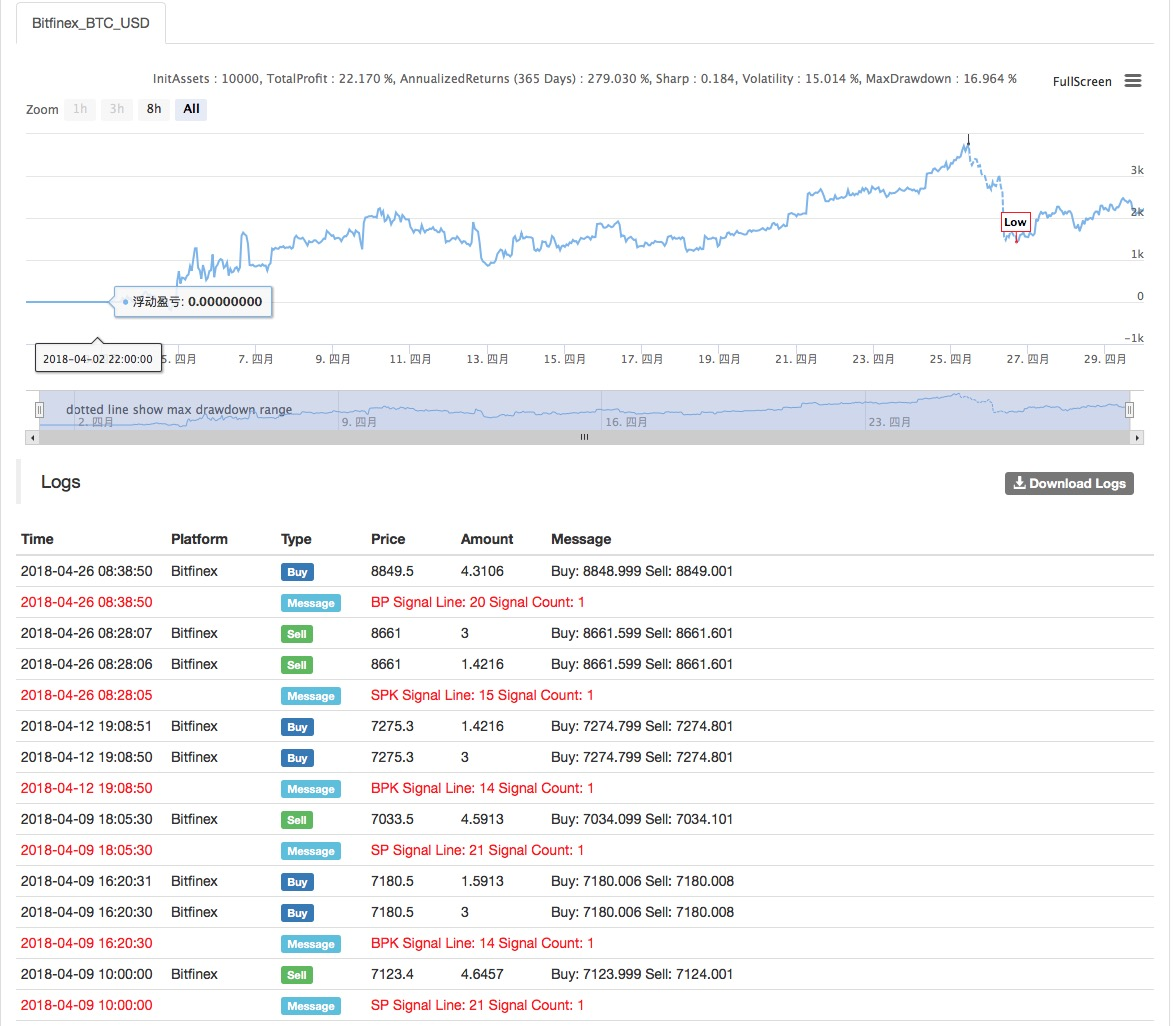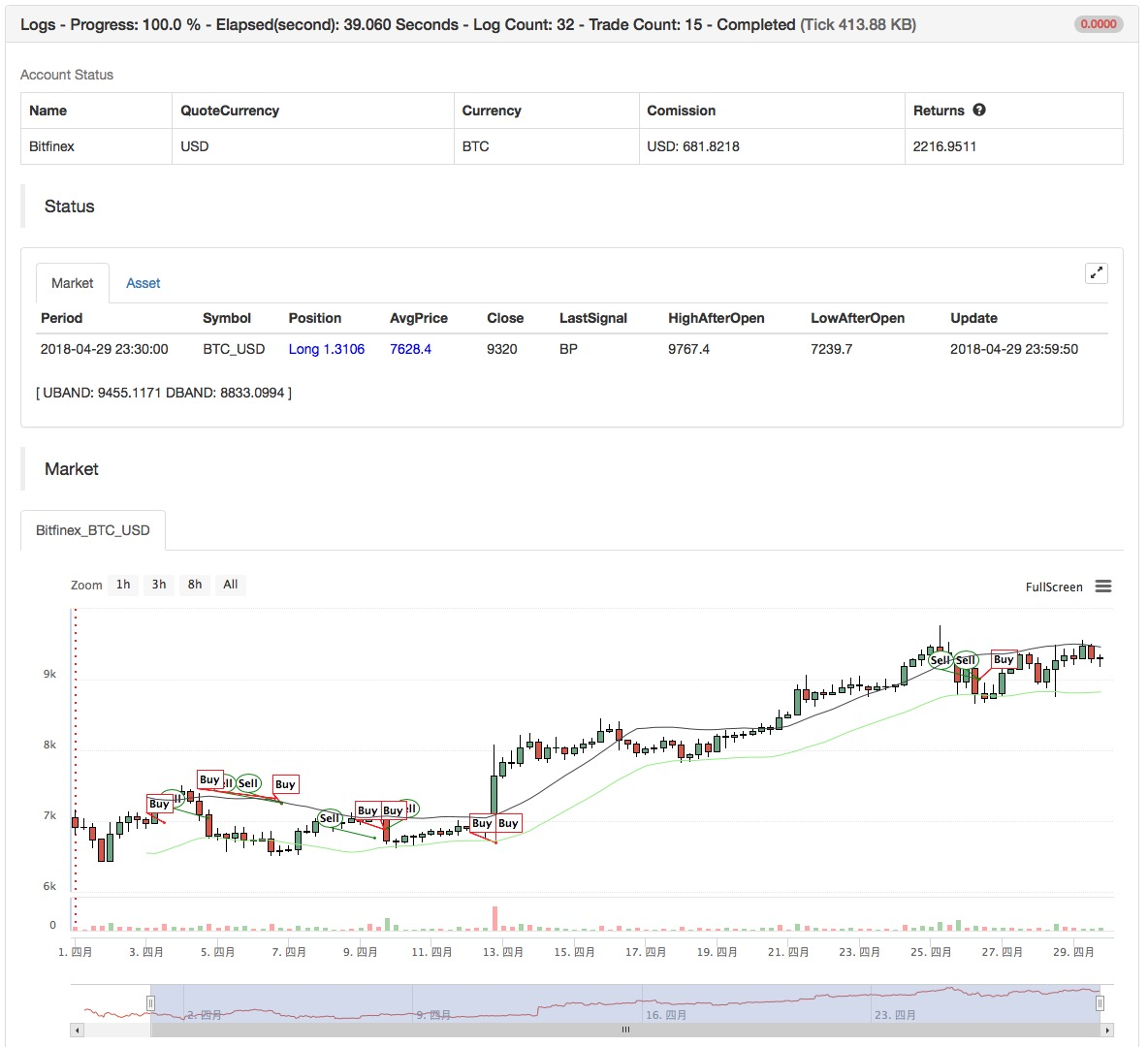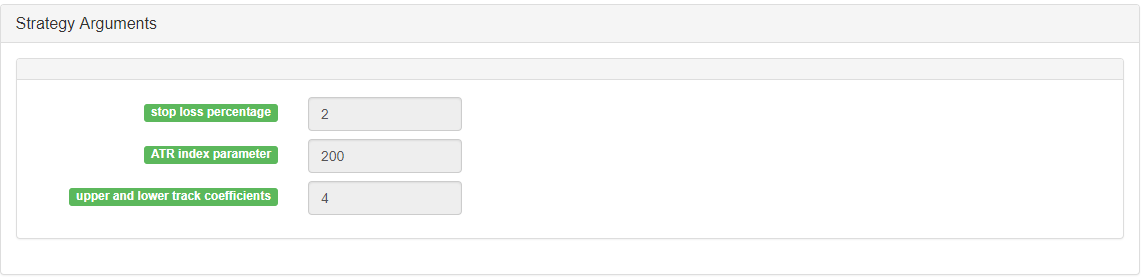# ATR Channel strategy Implemented on crypto market

Author: , Created: 2020-08-21 19:29:51, Updated: 2020-08-21 19:31:10

Strategy name: Channel strategy based on ATR volatility index

Strategy idea: Channel Adaptive Strategy, Fixed Stop + Floating Stop

Data Cycle: Multi-Cycle• Main chart: Draw UBAND, formula: UBAND ^^ MAC + MATR; Draw DBAND, formula: DBAND ^^ MAC-MATR;

• Secondary chart: none``````(*backtest
start: 2018-06-01 00:00:00
end: 2018-07-01 00:00:00
period: 1h
exchanges: [{"eid":"Futures_OKCoin","currency":"BTC_USD"}]
*)
​
TR1:=MAX(MAX((HIGH-LOW),ABS(REF(CLOSE,1)-HIGH)),ABS(REF(CLOSE,1)-LOW));
ATR:=MA(TR1,N);
MAC:=MA(C,N);
UBAND^^MAC+M*ATR;
DBAND^^MAC-M*ATR;
H>=HHV(H,N),BPK;
L<=LLV(L,N),SPK;
(H>=HHV(H,M*N) OR C<=UBAND) AND BKHIGH>=BKPRICE*(1+M*SLOSS*0.01),SP;
(L<=LLV(L,M*N) OR C>=DBAND) AND SKLOW<=SKPRICE*(1-M*SLOSS*0.01),BP;
// stop loss
C>=SKPRICE*(1+SLOSS*0.01),BP;
C<=BKPRICE*(1-SLOSS*0.01),SP;
AUTOFILTER;
``````

Source Code: https://www.fmz.com/strategy/128126

More